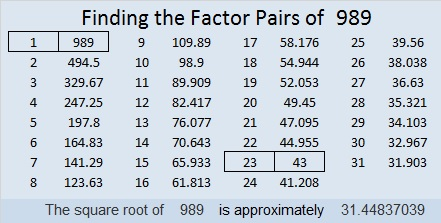# 1573 Ring Out Wild Bells

### Today’s Puzzle:

Here are the first two verses of Alfred Lord Tennyson’s Ring Out Wild Bells:

Ring out, wild bells, to the wild sky,
The flying cloud, the frosty light;
The year is dying in the night;
Ring out, wild bells, and let him die.

Ring out the old, ring in the new,
Ring, happy bells, across the snow:
The year is going, let him go;
Ring out the false, ring in the true.

I think we are all ready for 2020 to “die” so we can ring in 2021. You can get started with this bell-shaped puzzle. Use logic to find its unique solution.Print the puzzles or type the solution in this excel file: 12 Factors 1558-1573

### Factors of 1573:

This is my 1573rd post, so let’s find the factors of 1573.

• 1573 is a composite number.
• Prime factorization: 1573 = 11 × 11 × 13, which can be written 1573 = 11² × 13.
• 1573 has at least one exponent greater than 1 in its prime factorization so √1573 can be simplified. Taking the factor pair from the factor pair table below with the largest square number factor, we get √1573 = (√121)(√13) = 11√13.
• The exponents in the prime factorization are 2 and 1. Adding one to each exponent and multiplying we get (2 + 1)(1 + 1) = 3 × 2 = 6. Therefore 1573 has exactly 6 factors.
• The factors of 1573 are outlined with their factor pair partners in the graphic below.### More about the number 1573:

1573 is the sum of two squares:
33² + 22² = 1573.

1573 is the hypotenuse of a Pythagorean triple:
605-1452-1573, calculated from 33² – 22², 2(33)(22), 33² + 22².
It is also (5-12-13) times 121.

# 989 Christmas Bells at Eventide

I made this puzzle with silver clues to look like a bell. I thought dark blue looked best as a background color with the silver numbers. The puzzle reminds me of a bell in the evening. For the fun of it, I googled Christmas Bells in the evening to see if any poems or songs came up.Print the puzzles or type the solution in this excel file: 10-factors-986-992

I was very surprised that Google found a very old song called Christmas Bells at Eventide. I did not know that such a song existed. Eventide means the same thing as evening. You can listen to the song below.

This is my 989th post.

The number 989 is a palindrome in base 10. What about any other bases?
It’s 373 in BASE 17 because 3(17²) + 7(17) + 3(1) = 989,
252 in BASE 21 because 2(21²) + 5(21) + 2(1) = 989, and
1C1 in BASE 26 (C is 12 base 10) because 1(26²) + 12(26) + 1(1) = 989

• 989 is a composite number.
• Prime factorization: 989 = 23 × 43
• The exponents in the prime factorization are 1 and 1. Adding one to each and multiplying we get (1 + 1)(1 + 1) = 2 × 2 = 4. Therefore 989 has exactly 4 factors.
• Factors of 989: 1, 23, 43, 989
• Factor pairs: 989 = 1 × 989 or 23 × 43
• 989 has no square factors that allow its square root to be simplified. √989 ≈ 31.44837